# Can LabVIEW C? - Example 2: More Complex Programming Concepts

Publish Date: Oct 02, 2012 | 26 Ratings | 2.77 out of 5 | Print | 1 Customer Review | Submit your review

This set of examples (Teachers Assistant.vi and TeachersAssistant.c), linked below, is designed to illustrate the concepts of data structures, code modularization, file I/O, and data analysis in C and in LabVIEW. The application allows a teacher to enter and store student names, social security numbers, and grades, and then transfer this information to and from a file. These examples also perform some data analysis, calculating each student’s final grade, the class mean, and the class standard deviation. As in the previous example, the C program controls execution using a While Loop and a Switch-Case Structure and the LabVIEW program controls execution using a While Loop and a Case structure. Figure 12 and Figure 13 show the user interfaces for the C and LabVIEW examples.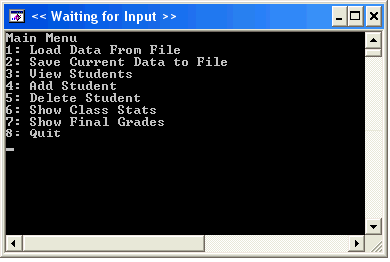Figure 12. C Standard Output Window for Teachers Assistant.c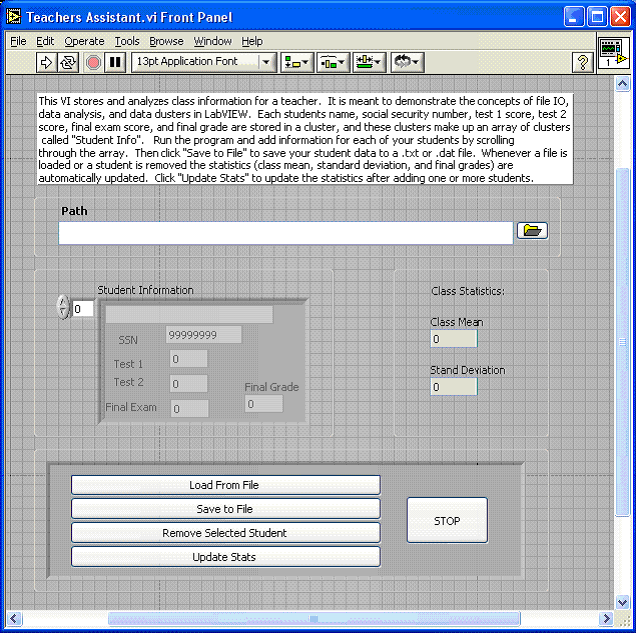Figure 13. LabVIEW Graphical User Interface for Teachers Assistant VI

### 1. Storing Data in Structures and Clusters

Both programs store data in similar ways. The C example uses a structure to store each student’s information. The student’s name is represented as a character array, the social security number as an integer, and the four grades as floating-point numbers. The LabVIEW example uses a cluster to store a student’s information. A LabVIEW cluster is very similar to a structure in C. It stores data of various types in one data structure. In LabVIEW, the student's name is represented as a string, the social security number as a 32-bit integer, and the four test grades as double-precision floating-point numbers.

The C program uses an array of structures to store data for all students. Identically, the LabVIEW program stores the information for all students in an array of clusters. Figure 14 shows the code defining the C structure, along with the front panel representation of the LabVIEW array of clusters.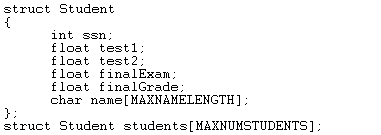Figure 14. C Definition of the “Student” Structure and Declaration of an Array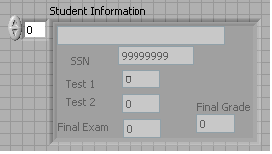Figure 15. LabVIEW Front Panel Image of an Array of Clusters

The representation of a numeric control can be specified from the property page for the control. A developer simply clicks the Representation icon and selects from twelve different numeric types. Figure 16 shows the property page for the Test 1 numeric control.Figure 16. Choosing a Numeric's Representation Using the Property Page in LabVIEW

A C programmer must access individual elements of the array and individual data members of a structure. When this occurs, an element can be extracted from an array using indexing and a data member can be unbundled from the structure using a period. Figure 17 shows the code from the C example that extracts each data member of index “i” from the array of structures.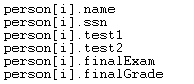Figure 17. C Code for Indexing the Array and Extracting Elements From the Structure

The process of accessing data is very similar in LabVIEW. To access an element of an array, a developer can use the Index Array function. This is functionally equivalent to indexing an array using brackets in C. To extract a specific element from the cluster, a developer can use the Unbundle function or Unbundle By Name function. Figure 18 below is an example of LabVIEW code indexing out a cluster from the array at index “i” and unbundling the elements of the cluster. Note that the LabVIEW code follows the concept of data flow by passing data in and out of VIs using wires.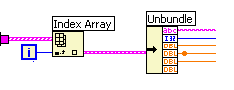Figure 18. LabVIEW Code for Indexing the Array and Extracting the Data Members from the Structure

### 2. Modularizing Code in Functions and SubVIs

Similarities between LabVIEW and C can also be found when examining how code is modularized and executed. A C programmer who is familiar with function calls will be very comfortable calling subVIs in LabVIEW. The C example stores subroutines as functions, each performing a specific task. These functions may have a return value and can be passed parameters. In the C example, all functions are passed the Student array of structures by reference. The loadFile and saveToFile functions are also passed a FILE handle.

A LabVIEW developer can handle code modularization in the same way, placing subroutines in separate VIs that can be called as subVIs. There is an equivalent LabVIEW subVI for many of the C example functions. Just as parameters are passed to C functions, inputs and outputs can be passed to any LabVIEW subVI. The color of the wire indicates the type of the data. In the above diagram, pink indicates a string, blue indicates an integer, and orange indicates a floating-point numeric value. LabVIEW also automatically generates extensive documentation for subVIs a developer creates. Figure 19 below is a function prototype for the saveToFile() C function. Figure 20 is a small part of the LabVIEW generated documentation for the Save VI.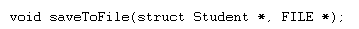Figure 19. C Function Prototype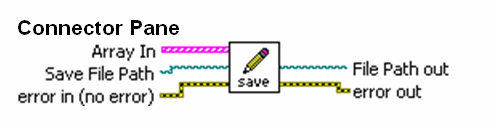Figure 20. Part of the LabVIEW Generated Documentation for the Save VI Displaying the Inputs and Outputs

Setting the connection terminals for a subVI is accomplished by using the wiring tool to connect a terminal on the VI’s connector pane to a front panel control for an input and to a front panel indicator for an output. Once a subVI is created, LabVIEW makes it easy for a developer to determine what data he needs to pass in and out of a subVI by selecting Help»Show Context Help to view a help description and a diagram of the connection terminals. Figure 21 displays the Save VI's front panel demonstrating how to wire connectors from a front panel.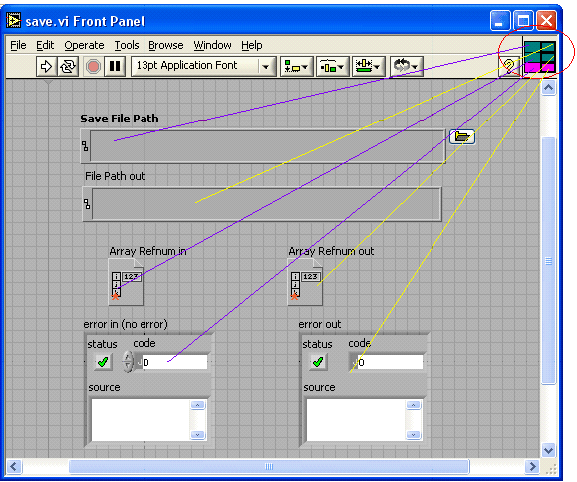Figure 21. Associating Data with Connector Pane Terminals from the Save VI

The C example passes the array of structures by reference from the main function to all the other functions. Passing by reference benefits performance because another copy of the data does not need to be made. As discussed in the previous example, LabVIEW appears to pass the array by value but in practice is passing by reference. LabVIEW does all modifications on the data inplace and does not have to make another copy of the array.

### 3. File I/O

LabVIEW also includes  many built-in file input/output (I/O) and string parsing functions. File I/O is yet another operation that can be approached similarly in C. In the loadFile() function, the C example uses the fgets() function inside of a While Loop in order to read lines from a file one at a time. The C example then uses the scanf() function to parse the string, scanning out the necessary data. Figure 22 displays this code.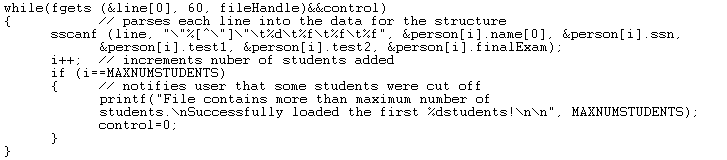Figure 22. C Code for Reading Lines from a File and Scanning the Data

The LabVIEW example’s routine for loading data from a file is structurally identical to the example in C. In place of the fgets() function used in C, a LabVIEW developer can use the built-in Read Lines From File VI. This VI also reads data from a file one line at a time. The LabVIEW example also places this VI inside of a While Loop and stops the execution of the While Loop when the end of the file has been reached.

Once a line has been read from the file, the string is then passed to the Scan From String function. Much like the scanf() C function, this VI parses the string and scans out the necessary data. The C scanf() function and the LabVIEW Scan From String function even take similar inputs. The format string, which is a regular expression indicating how the string should be scanned, is nearly identical for the two languages. Once the data has been scanned, it is bundled into a cluster and passed out of the While Loop.

This leads into another time-saving feature of LabVIEW. Using the auto-indexing feature in LabVIEW, wiring one cluster from inside of a While Loop to the outer wall can automatically build an array. This feature can also be disabled if required. Figure 23 shows the block diagram code that reads lines of data from a file and parses the strings.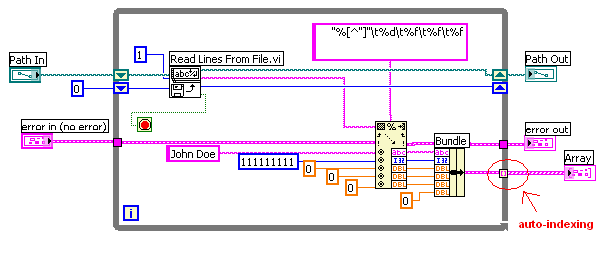Figure 23. LabVIEW Code for Reading Lines From a File and Scanning the Data

Both programs also give the user the option to remove a student from memory by removing an element from the array of clusters. In the C example, the array position of the student to be removed is determined by scrolling through the array and comparing either names or social security numbers. Once the position index is determined, the student is removed by once again scrolling through the array and decrementing the index position of every student above the index position. The size of the array is also decremented.

A developer could easily duplicate this process in LabVIEW. However, this is a case where LabVIEW offers a faster solution using built-in functions. The Teacher's Assistant VI uses the built-in Delete From Array VI to simply and efficiently remove a student from the array at the selected index. Figure 24 and 25 show the C and LabVIEW code that actually removes a student from the array.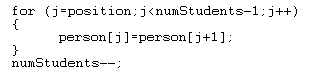Figure 24. C Code to Remove the Student at Index “j”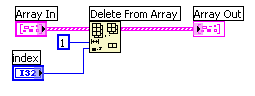Figure 25. LabVIEW Code to Remove a Student at a Given Index

### 4. Analyzing Data

C and LabVIEW are also both capable performing data analysis.  In fact, scientific data analysis is one of the core strengths of LabVIEW. The analysis is kept relatively simple in these examples. Both examples calculate each student’s final grade, as well as the class mean and standard deviation. The C and LabVIEW examples calculate the final grades identically by weighing and averaging the student's test grades inside of a For Loop. However, LabVIEW offers the developer an advantage when calculating the standard deviation and mean. While the C example uses a loop and some equations, the LabVIEW example simply passes the array of final grades into the built-in Standard Deviation and Variance VI. This VI returns both the mean and standard deviation of the array.

Although built-in analysis VIs, such as the Standard Deviation and Variance VI can save a developer valuable time, they in no way limit developer flexibility. If you examine the block diagram of the Standard Deviation and Variance VI, you will notice that the subroutine itself was written in LabVIEW. The Standard Deviation and Variance VI is a great illustration of the strengths of LabVIEW: completely capable of low-level programming while at the same time being an easy to use, time-saving software environment. The Standard Deviation and Variance VI is just one of hundreds of high-level built-in LabVIEW analysis VIs. Figures 26 and 27 show the analysis code used to calculate the statistics in both C and LabVIEW. Figure 28 shows the block diagram of the built-in Standard Deviation and Variance VI, which is used in the LabVIEW code for the example below.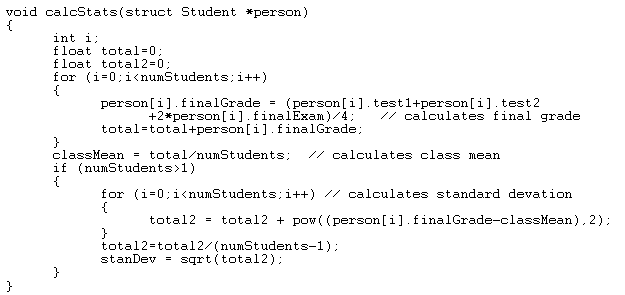Figure 26. C Function that Calculates the Statistics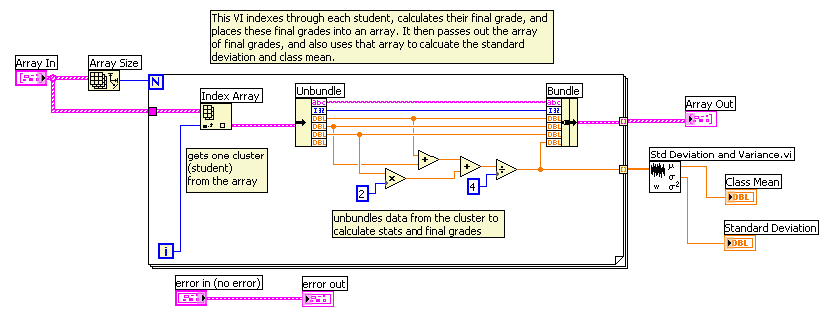Figure 27. LabVIEW Code From the Calculate VI That Calculates the Statistics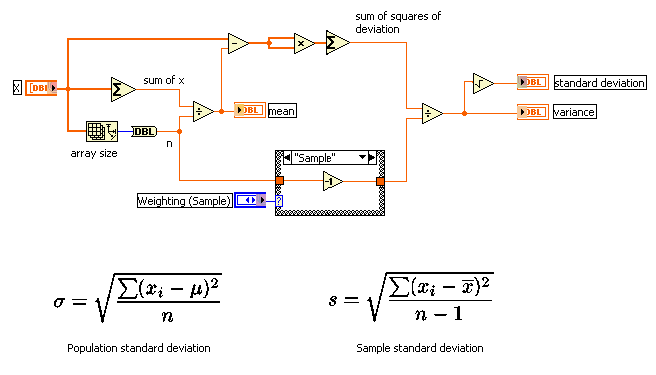Figure 28. Block Diagram of the Built-In Standard Deviation and Variance VI

Customer Reviews
1 Review | Submit your review

scaning from string  - Nov 11, 2004

scaning from string is well defined as also compared with the c language . i think this is a perfect exmaple of defining complex string scaning through labview

### Ratings

Rate this document